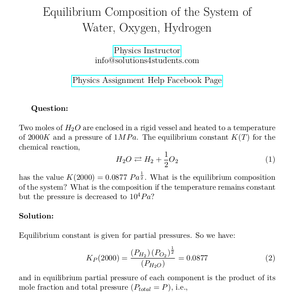Solved Problems in Physics, Physical Chemistry, and Computer Simulation Projects for College and Graduate Students.# Equilibrium Composition of the System of Water, Oxygen, Hydrogen

Regular price
\$4.00
Sale price
\$4.00
Tax included.

Question:

Two moles of H2O are enclosed in a rigid vessel and heated to a temperature of 2000K and a pressure of 1Mpa. The equilibrium constant K(T) for the chemical reaction,

H2O ⮂ H2 + 1/2 H2

has the value K(2000)=0.0877 Pa1/2. what is the equilibrium composition of the system? What is the composition if the temperature remains constant but the pressure is decreased to 104 Pa?

By purchasing this product, you will get the step by step solution of the above problem in pdf format and the corresponding latex file where you can edit the solution, and a Matlab/Octave code that is the numerical solution of a equation obtained in the solution, by Newton-Raphson method. I am also available to help  you with any possible question you may have.